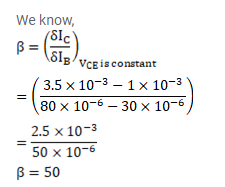# When the base current in a transistor is changed from 30μA to 80 μA,

Question:

When the base current in a transistor is changed from $30 \mu \mathrm{A}$ to $80 \mu \mathrm{A}$, the collector is changed from $1.0 \mathrm{~mA}$ to $3.5 \mathrm{~mA}$. find the current gain $\beta$.

Solution: